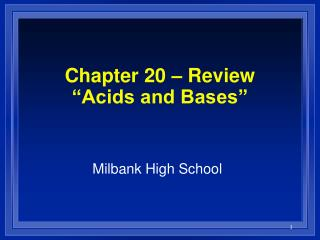DownloadDownload PresentationChapter 20 – Review “Acids and Bases”

# Chapter 20 – Review “Acids and Bases”

Télécharger la présentation## Chapter 20 – Review “Acids and Bases”

- - - - - - - - - - - - - - - - - - - - - - - - - - - E N D - - - - - - - - - - - - - - - - - - - - - - - - - - -
##### Presentation Transcript

1. Chapter 20 – Review“Acids and Bases” Milbank High School

2. Chapter 20 - Review • When an acid reacts with a base, what compound(s) are formed? • What is the name of H2SO3? • What is the formula for phosphoric acid? • Give some properties of acids. • Give some properties of bases.

3. Chapter 20 - Review • If the hydrogen ion concentration is 10-10 M, is the solution acidic, alkaline, or neutral? • If the [H1+] in a solution is 1 x 10-1 mol/L, what is the [OH1-]?

4. Chapter 20 - Review • If the hydrogen ion concentration is 10-7 M, what is the pH of the solution? • If the hydroxide ion concentration is 10-10 M, what is the pH of the solution? • If the pH is 9, what is the concentration of the hydroxide ion?

5. Chapter 20 - Review • For a solution to be classified as acidic, the hydrogen-ion concentration must be _____ than the hydroxide-ion concentration. • If the pH is 6, what is the concentration of the hydrogen ion?

6. Chapter 20 - Review • A solution in which the hydroxide ion concentration is 1 x 10-4 M is ______. • A solution in which the hydroxide ion concentration is 1 x 10-8 M is ______. • The formula of the hydrogen ion is often written as ______.

7. Chapter 20 - Review • The products of self-ionization of water are _____. • Which is most basic: a) [OH1-] = 1 x 10-4 M, or b) [H1+] = 1 x 10-11 M? • In a neutral solution, the [H1+] is ______. • What is pH?

8. Chapter 20 - Review • In the reaction of water with hydrogen ions, which compound is the Lewis base? • What is an acid according to Arrhenius? • What type of acid (mono-, di-, or triprotic) is sulfuric acid?

9. Chapter 20 - Review • Which is an Arrhenius base: a) LiOH, or b) CH3COOH? • What is an acid, according to Bronsted? • Which of the following is a Bronsted-Lowry base, but not an Arrhenius base: a) sodium hydroxide, or b) ammonia?

10. Chapter 20 - Review • Which compound can act as both a Bronsted-Lowry acid and a Bronsted-Lowry base: a) water, or b) sodium chloride? • Which type of solution is one with a pH of 8?

11. Chapter 20 - Review • What is transferred between a conjugate acid-base pair? • What is the term used to describe a substance that can be both an acid and a base? • In the reaction: NH41+ + H2O  NH3 + H3O1+, water is acting as a(n) ______.

12. Chapter 20 - Review • A Lewis acid is a substance that can _____. • What is the acid dissociation constant of a weak acid, if a concentration of 0.3 M gives a hydrogen ion concentration of 0.001 M?

13. Chapter 20 - Review • What is the hydrogen ion concentration, if the acid dissociation constant is 1 x 10-6 M and the acid concentration is 0.01 M? • What characterizes a “strong” acid or base?

14. Chapter 20 - Review • A 0.12 M solution of an acid that ionizes only slightly in solution would be termed ___ and ___. • A 12.0 M solution of an acid that ionizes completely in solution would be termed ___ and ____.

15. Chapter 21 – Review“Neutralization” Milbank High School

16. Chapter 21 - Review • What products result from a neutralization reaction? • What is obtained when phosphoric acid and magnesium hydroxide are mixed? • What are the products of the reaction of one mole of Mg(OH)2 and one mole of H2SO4?

17. Chapter 21 - Review • What is the gram-equivalent mass of magnesium hydroxide if magnesium hydroxide has a gram-molecular mass of 58.0 g? • What is the purpose of a titration?

18. Chapter 21 - Review • How many equivalents per mole are there in nitric acid? • What is the gram-equivalent mass of chromic acid, H2CrO4?